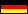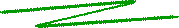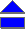The Posten container problemA forwarding agent shall supply various articles by container to a supermarket. The container has given capacities of weigt and of volume, whereas the articles have fixed weight, volume, and value per item. How many items of each article should the forwarding agent pack into a container such that the container contents has maximum value under the constraint that given minimum quantities per article are to be supplied?

Let for instance the articles A, B, C as well as the capacity limits of the container be given according to the following table.

article
 volume (m3/item)
 weight (kg/item)
demand
 price (k€/item)
A 6 3 1 10
B 3 2 1 8
C 1 1 1 2
capacity 15 8 - -

Article A thus has a volume of 6 m3 and a weight of 3 kg per item, and must be supplied at least once; its value is 10 000 €.

With Dynamic Programming, a method of Operations Research, the problem is solved as a multistage decision problem. However, you may solve this small example above by simple enumeration:

1 - 2 - 1     (read: 1x A, 2x B, 1x C),

with a value of 28 k€. Please press the start button to solve an arbitrary Posten problem (You can determine the number of articles, but at present the number of columns cannot be changed):

===================
 © de Vries 2002